Home | | Engineering Physics I | Bending of Beams

# Bending of Beams

Beams: A beam is defined as a rod or bar. Circular or rectangular of uniform cross section whose length is very much greater than its other dimensions, such as breadth and thickness. It is commonly used in the construction of bridges to support roofs of the buildings etc. Since the length of the beam is much greater than its other dimensions the shearing stresses are very small.

BENDING OF BEAMS

1 Beams: A beam is defined as a rod or bar. Circular or rectangular of uniform cross section whose length is very much greater than its other dimensions, such as breadth and thickness. It is commonly used in the construction of bridges to support roofs of the buildings etc. Since the length of the beam is much greater than its other dimensions the shearing stresses are very small.

Assumptions:

While studying about the bending of beams, the following assumptions have to be made.

1.           The length of the beam should be large compared to other dimensions.

2.           The load(forces) applied should be large compared to the weight of the beam

3.           The cross section of the beam remains constant and hence the geometrical moment of inertia ig also remains constant

4.           The shearing stresses are negligible

5.           The curvature of the beam is very small

2 Bending of a Beam and neutral axis

Let us consider a beam of uniform rectangular cross section in the figure. A beam may be assumed to consist of a number  of parallel longitudinal metallic fibers placed one over the other and are called as filaments as shown in the figure.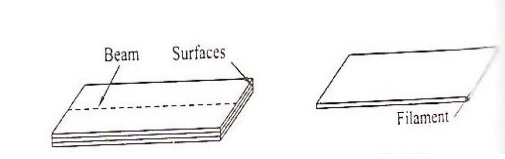Let the beam be subjected to deforming forces as its end as shown in the figure. Due to the deforming force the beam bends. We know the beam consist of many filaments. Let us consider a filament AB at the beam. It is found that the filaments(layers) lying above AB gets elongated, while the filaments lying below AB gets compressed. Therefore the filaments i.e layer AB which remains unaltered is taken ass the reference axis called neutral axis and the plane is called neutral plane. Further, the deformation of any filaments can be measured with reference to the neutral axis.3 EXPRESSION FOR BENDING MOMENT

Let us consider a beam under the action of deforming forces. The beam bends into a circular arc as shown in the figure. Let AB be the neutral axis of the beam. Here the filaments above AB are elongated and the filaments below AB are compressed. The filament AB remains unchanged.Let PQ be the chosen from the neutral axis. If R is the radius of curvature of the neutral axis and ᶿ is the angle subtended by it at its center of curvature’C’

Then we can write original length

PQ=Rᶿ  ………………………………………………………. 1

Let us consider a filament P’Q’ at a distance ‘X’ from the neutral axis.

We can write extended length

P’Q’=(R+x)ᶿ  ………………………………………………2

From equations 1 and 2 we have,

Increase in length=P’Q’-PQ

On increase in its length=(R=x)θ-Rθ

Increase in length=xθ  …………………………………….3

We know linear strain=increase in length\original length

Linear strain=xθ\Rθ=x\R  ………………………………4

We know, the youngs modulus of the material

Y=stress\linear strain

Or

stress=y*linear strain …………………….5

Substituting 4 in 5, we have

Stress=Yx\R

If δA is the area of cross section of the filament P’Q’, then,

The tensile force on the area δA=stress*Area

Ie. Tensile force=(Yx\R ).δa

We know the memont of force= force*Perpendicular distance

Moment of the tensile force about the neutral axis AB

orThe moment of force acting on both the upper and lower halves of the neutral axis can be got by summing all the moments of tensile and compressive forces about the neutral axis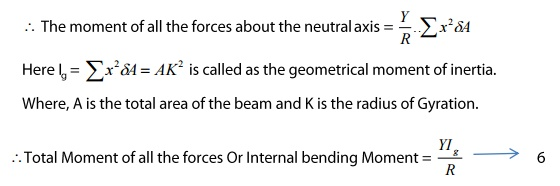SPECIAL CASES

a)          Rectangular Cross section

If ‘b’ is the breadth and ‘d’ is the thickness of the beam, themb)          Circular Cross Section4 NON-UNIFORM BENDING-DEPRESSION OF THE MID POINT OF A BEAM LAODED AT THE MIDDLE THEORY

Let us consider a beam of length ‘l’ (distance between the two knife edges) supported on the two knife edges A and B as shown in the figure. The load of weight ‘W’ is suspended at the centre ‘C’. It is found that the beam bends and the maximum displacement is at the point ‘D’Where the load is given.

Due to the load (W) applied, at the middle of the beam the reaction W/2 is acted vertically upwards at each knife edges. The bending is celled Non-Uniform bendingThe beam may be considered as two cantilevers, whose free end carries a load of W/2 and fixed at the point ‘D’.

Hence we can say the elevation of A above D as the depression below ‘A’. We know the depression of a cantileverTherefore substituting the value l and l/2 and was W/2 in the expression for the depression of the cantilever we have5 UNIFORM BENDING-ELEVATION AT THE CENTER OF THE BEAM LOADED AT BOTH THE ENDS THEORY:

Let us consider a beam of negligible mass, supported symmetrically on the two knife edges A and B as shown. Let the length between A and B is’l’. Let equal weights W; be added to either end of the beam C and D.Let CA=BD

Due to load applied the beam bends from position F and e into an arc of a circle and produces as elevation ‘x’ from position F and E. Let ‘W’ be the reaction produced at the points A and B acts vertically upwards as shown in figure.

Consider a point ‘P’ on the cross section of the beam. Then the forces acting on the part PC of the beam are

a)           Force W at ‘C’ and

b)          Reaction W at A as shown in the figureHere the clockwise moment is taken as negative and anticlockwise moment is taken as positive.

External bending moment about P can be written asExternal bending moment = Internal bending moment

We can write Equation 1 = Equation 2Since for a given load (W) Y, Ig and R are constant the bending is called Bending. Here it is found that the elevation ‘x’ forms an arc of the circle of radius ‘R’, as shown in the figure.6 DEPRESSION OF A CANTILEVER WHEN LOADED AT ITS END CANTILEVER:

A cantilever is a beam fixed horizontally at one end loaded to the other end.

THEORY:

Let us consider a beam fixed at one end and loaded at its other end as shown in the figure.

Due to load applied at the free end, a couple is created between the two forces

a. Force (load ‘W’) applied at the free end towards downward direction and

b. Reaction(R) acting in the upward direction at the supporting end

The external bending couple tends to bend in the clockwise direction. But since one end of the beam is fixed, the beam cannot rotate. Therefore external bending couple must be balanced by another equal and opposite couple, created due to elastic nature of the body

i.e. called as internal beading moment.

Under equilibrium condition

External bending moment = Internal bending Moment

7 DEPRESSION OF A CANTILEVER – LOADED AT ITS ENDS

THEORY:

LET ‘I’ be the length of the cantilever OA fixed at ‘O’. Let ‘W’ be the weight suspended (loaded) at the free end of the cantilever. Due to the load applied the cantilever moves to a new position OA’ as shown in this figure.Let us consider an element PQ of the beam of length dx, at a distance OP=x from the fixed end. Let ‘C’ be the center of curvature of the element PQ and let ‘R’ be the radius of the curvature.

Due to the load applied at the free end of the Cantilever, an external couple (Distance between the two equal and opposite forces) is (l-x).We know under thermal equilibrium

External bending moment = Internal bending Moment

Therefore, we can write Eqn 1 = Eqn 2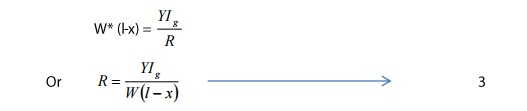Two tangents are drawn at points P and Q, which meet the vertical line AA’ at T and S respectively

Let the smallest depression produced from T to S = dy and

Let the angle between the two tangents = dƟ

Then we can write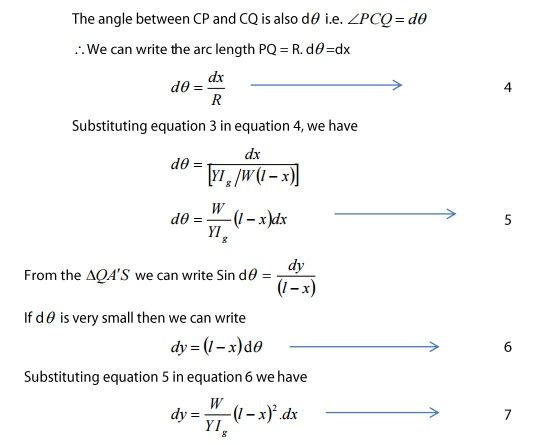Total depression at the end of the cantilever can be derived by integrating the equation 7 within the limits ‘0’ to ‘1’.SPECIAL CASES:

a. RECTANGULAR CROSS SECTION

If ‘b’ is the breadth and ‘d’ is the thickness of the beam then we knowSubstituting the value of Ig in equation 8 we can write

The depression produced at the free end for a rectangular cross sectionb. CIRCULAR CROSS SECTION

If ‘r’ is the radius of the circular cross section, thenSubstituting the value of Ig in equation 8 we can write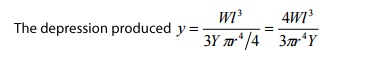Study Material, Lecturing Notes, Assignment, Reference, Wiki description explanation, brief detail
Physics : Properties of Mater and Thermal Physics : Bending of Beams |